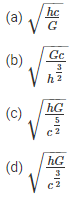# Physical World And Measurement MCQs

NEET  Physics is the scoring paper in the medical entrance examination. Here, you will discover the NEET Physics MCQ Questions for all Concepts as per the latest syllabus. Practice more on a regular basis with these NEET Physics objective questions on air pollution and improve your subject knowledge & problem-solving skills along with time management. NEET Physics Physics Physical World And Measurement Multiple Choice Questions make you feel confident in answering the question in the exam & increases your scores to high.

## MCQ on Physical World And Measurement

1. How many numbers of base SI units are there?
(a) 5
(b) 10
(c) 7
(d) 9

2. What is the percentage error in the measurement of the kinetic energy of a body, if there is a positive error of 50% in the speed of the body
(a) 125%
(b) 30%
(c) 50%
(d) 100%

3. What is the dimensional formula of torque and energy
(a) [ML-3T-2] and [MLT-2]
(b) [ML2T-2] and [MLT-2]
(c) [ML2T-2] and [ML2T2]
(d) [MLT2] and [ML2T2]

4. Find the relative error of y, if y=x2
(a) $$\frac{\triangle x}{x}$$
(b) $$\frac{2 \triangle x}{x}$$
(c) $$\frac{(\wedge x)^{2}}{x}$$
(d) (△x)2

Answer: (b) $$\frac{2 \triangle x}{x}$$

5. Identify the physical parameters that are having the same dimensions from the below-given list:
(i) Magnetic field
(ii) Energy density
(iii) Refractive index
(iv) Young’s modulus
(v) Dielectric constant
(a) (ii) and (iv)
(b) (i) and (v)
(c) (iii) and (iv)
(d) (iv) and (iii)

6. What is the angle between A and B, if $$\vec{A}+\vec{B}=\vec{C}$$ such that $$\vec{C}$$ is perpendicular to $$\vec{A}$$ and $$|\vec{A}|=|\vec{C}|$$
(a) π
(b) $$\frac{\pi}{2}$$
(c) $$\frac{\pi}{4}$$
(d) $$\frac{3 \pi}{4}$$

Answer: (d) $$\frac{3 \pi}{4}$$

7. Which the following is a dimensionless quantity
(a) Refractive index
(b) Gravitational constant
(c) Velocity
(d) Planck’s constant

8. Calculate the value of the dot product of the two vectors whose magnitudes are 3 units and 4 units and their resultant is 1 unit.
(a) +6 units
(b) 0
(c) -12 units
(d) -1 unit

9. From the given three fundamental constants, select the combination that gives the dimension of length
(i) Newton’s gravitational constant (G)
(ii) Speed of light in a vacuum (c)
(iii) Planck’s constant (h)Answer: (d) $$\sqrt{\frac{h G}{c^{\frac{3}{2}}}}$$

10. Following are the readings obtained while measuring the diameter of a wire by using the screw gauge0 mm is the main scale reading
52 divisions are the circular scale reading
What is the diameter of the wire if 1 mm on the main scale is equal to the 100 divisions on the circular scale
(a) 0.016 cm
(b) 0.052 cm
(c) 0.15 cm
(d) 0.05 cm